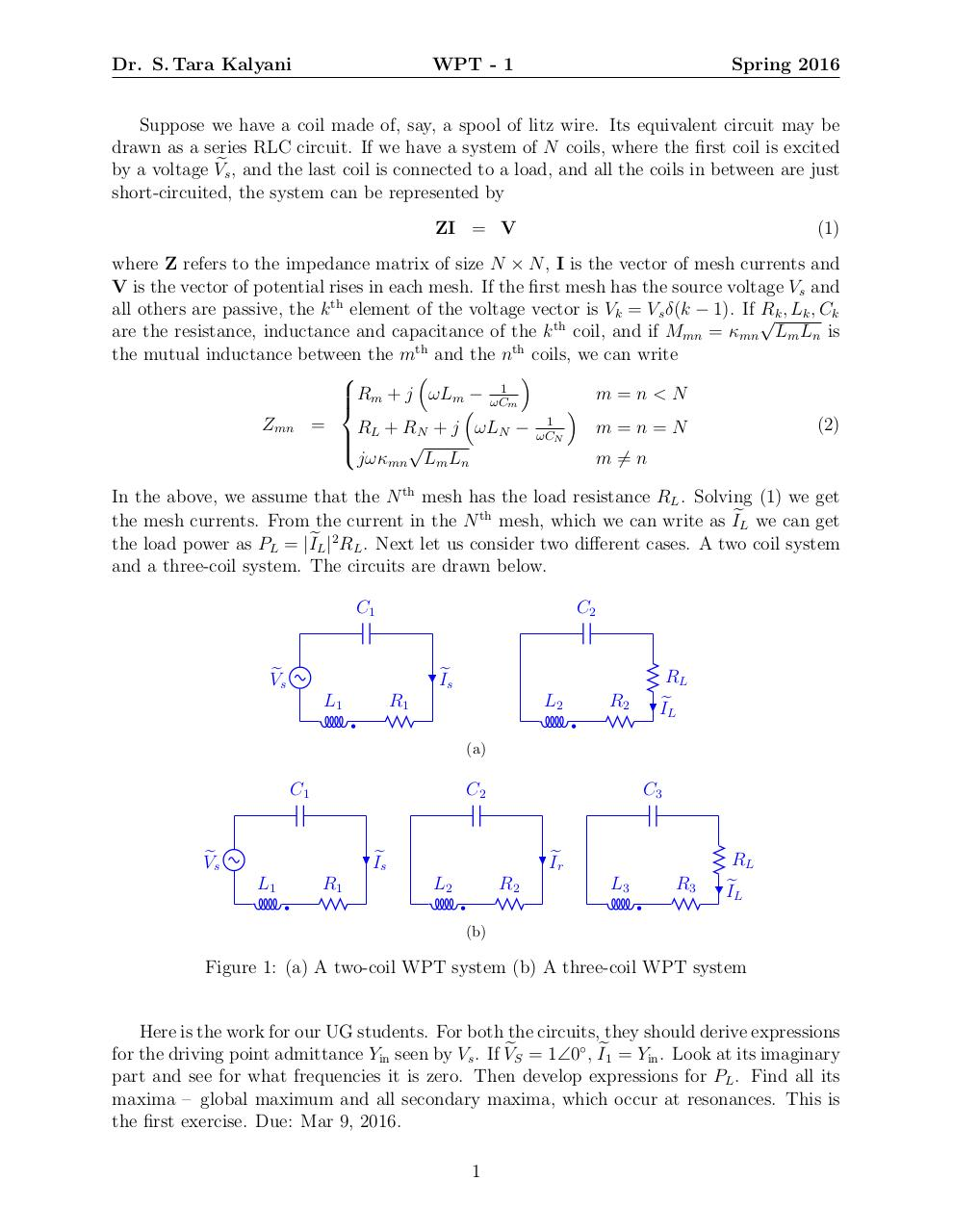wpt1 .pdf

File information

Original filename: wpt1.pdf

This PDF 1.5 document has been generated by TeX / pdfTeX-1.40.12, and has been sent on pdf-archive.com on 08/03/2016 at 10:54, from IP address 124.123.x.x. The current document download page has been viewed 357 times.
File size: 119 KB (1 page).
Privacy: public file

wpt1.pdf (PDF, 119 KB)

Document preview

Dr. S. Tara Kalyani

WPT - 1

Spring 2016

Suppose we have a coil made of, say, a spool of litz wire. Its equivalent circuit may be
drawn as a series RLC circuit. If we have a system of N coils, where the first coil is excited
by a voltage Ves , and the last coil is connected to a load, and all the coils in between are just
short-circuited, the system can be represented by
ZI = V

(1)

where Z refers to the impedance matrix of size N × N , I is the vector of mesh currents and
V is the vector of potential rises in each mesh. If the first mesh has the source voltage Vs and
all others are passive, the k th element of the voltage vector is Vk = Vs δ(k − 1). If √
Rk , Lk , Ck
th
are the resistance, inductance and capacitance of the k coil, and if Mmn = κmn Lm Ln is
the mutual inductance between the mth and the nth coils, we can write



1

m=n&lt;N

Rm + j ωLm− ωCm

Zmn =
(2)
RL + RN + j ωLN − ωC1N
m=n=N

jωκmn Lm Ln
m 6= n
In the above, we assume that the N th mesh has the load resistance RL . Solving (1) we get
the mesh currents. From the current in the N th mesh, which we can write as IeL we can get
the load power as PL = |IeL |2 RL . Next let us consider two different cases. A two coil system
and a three-coil system. The circuits are drawn below.
C1

C2

Ves

RL
IeL

Ies
L1

R1

L2

R2

(a)

C1

C2

Ves

C3

Ies
L1

R1

Ier
L2

R2

L3

R3

RL
IeL

(b)

Figure 1: (a) A two-coil WPT system (b) A three-coil WPT system

Here is the work for our UG students. For both the circuits, they should derive expressions
for the driving point admittance Yin seen by Vs . If VeS = 1∠0◦ , Ie1 = Yin . Look at its imaginary
part and see for what frequencies it is zero. Then develop expressions for PL . Find all its
maxima – global maximum and all secondary maxima, which occur at resonances. This is
the first exercise. Due: Mar 9, 2016.
1HTML Code

Copy the following HTML code to share your document on a Website or Blog

QR CodeRelated keywords